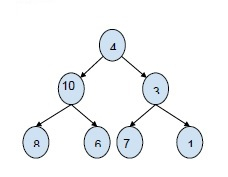# Print all the paths from root, with a specified sum in Binary tree in C++

In this problem, we are given a Binary tree and a sum S. And we have to find the path starting from root to any node of the tree, which gives the sum equal to the given sum.

InputSum = 14
Output : path : 4 10
4 3 7

To find the solution to this problem, we need to find the preorder traversal of the binary tree. And then find the path that adds up to the given sum.

## Example

Live Demo

#include<bits/stdc++.h>
using namespace std;
struct Node{
int key;
struct Node *left, *right;
};
Node* insertNode(int key){
Node* temp = new Node;
temp->key = key;
temp->left = temp->right = NULL;
return (temp);
}
void printPathsUtilSum(Node* curr_node, int sum, int
sum_so_far, vector<int> &path){
if (curr_node == NULL)
return;
sum_so_far += curr_node->key;
path.push_back(curr_node->key);
if (sum_so_far == sum ){
for (int i=0; i<path.size(); i++)
cout<<path[i]<<"\t";
cout<<endl;
}
if (curr_node->left != NULL)
printPathsUtilSum(curr_node->left, sum,
sum_so_far, path);
if (curr_node->right != NULL)
printPathsUtilSum(curr_node->right, sum,
sum_so_far, path);
path.pop_back();
}
void pathWithSum(Node *root, int sum){
vector<int> path;
printPathsUtilSum(root, sum, 0, path);
}
int main (){
Node *root = insertNode(4);
root->left = insertNode(10);
root->right = insertNode(3);
root->right->left = insertNode(7);
root->right->right = insertNode(1);
root->left->left = insertNode(8);
root->left->right = insertNode(6);
int sum = 14;
cout<<"Paths with the given sum are : "<<endl;
pathWithSum(root, sum);
return 0;
}

## Output

Paths with the given sum are −

4 10
4 3 7